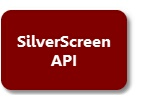﻿ Interactive Drawing Commands
SilverScreen Solid Modeler

# Interactive Drawing Commands# Interactive Drawing Commands# IDRAW Command (Interactive Drawing)

All of the interactive drawing commands can be invoked with the IDRAW command. Here are the most common formats for the command:

 idraw arc {angles|chord|points}  idraw bezier {closed|open} {single|double}  idraw circle {diameter|radius points}  idraw donut {diameter|radius} between  idraw ellipse  idraw hole  idraw lines {single|multiple}  idraw polygon {common|isosceles|quad|rectangle|triangle}  idraw polygon equilateral {edge vertex}  idraw polygon linear {center|left|right}  idraw polygon star radius  idraw polygon wedge {angles|chord|points}  idraw point common  idraw point {triad|xy-plane|yz_plane|zx-plane} length  idraw polyline  idraw spline {open|closed}

Since the user has the opportunity to escape from any of these com­mands, a program must be able to detect whether the user successfully completed the task. Here is one strategy for accomplishing this: create a new (empty) object; IDRAW; check to see if the object is empty. The code might appear similar to the following example:

 C / C++ Code OBJECT_NODE *obj;    ss_command ( "goto root" );  ss_command ( "create object temp#" );  ss_command ( "idraw polygon common" );  obj = (OBJECT_NODE *)get_bos (“\\temp#”);    if ( obj->first_prim )     ss_command ( "note The polygon was sucessfully drawn" );  else     ss_command ( "note The user aborted the drawing process" );